# Solving Equations by by Solving Equations 1 3

• Slides: 29Solving Equations by by Solving Equations 1 -3 Multiplying or Dividing Warm Up Lesson Presentation Lesson Quiz Holt Mc. Dougal Algebra 1Solving Equations by 1 -3 Multiplying or Dividing Warm Up Evaluate each expression. 1. (– 7)(2. 8) 19. 6 2. 0. 96 ÷ 6 0. 16 3. (– 9) 81 4. 1 5. 2 3 6. 1. 8 Holt Mc. Dougal Algebra 1Solving Equations by 1 -3 Multiplying or Dividing Objective Solve one-step equations in one variable by using multiplication or division. Holt Mc. Dougal Algebra 1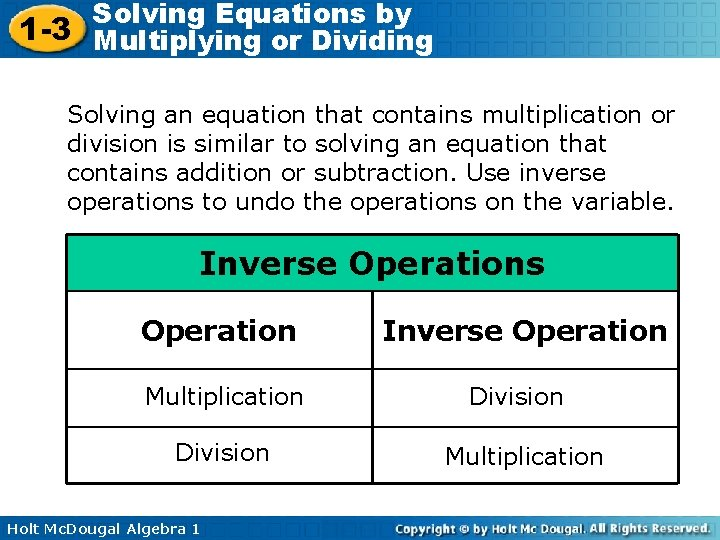Solving Equations by 1 -3 Multiplying or Dividing Solving an equation that contains multiplication or division is similar to solving an equation that contains addition or subtraction. Use inverse operations to undo the operations on the variable. Inverse Operations Operation Multiplication Division Holt Mc. Dougal Algebra 1 Inverse Operation Division Multiplication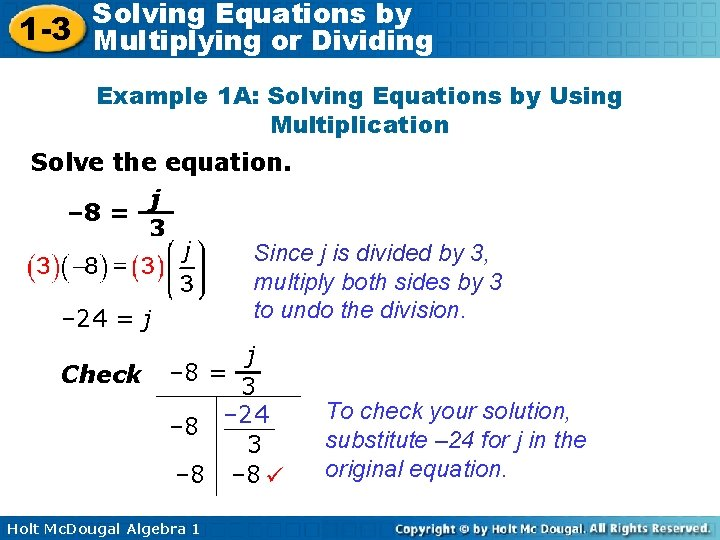Solving Equations by 1 -3 Multiplying or Dividing Example 1 A: Solving Equations by Using Multiplication Solve the equation. j – 8 = 3 Since j is divided by 3, multiply both sides by 3 to undo the division. – 24 = j Check j – 8 = 3 – 8 – 24 3 – 8 Holt Mc. Dougal Algebra 1 To check your solution, substitute – 24 for j in the original equation.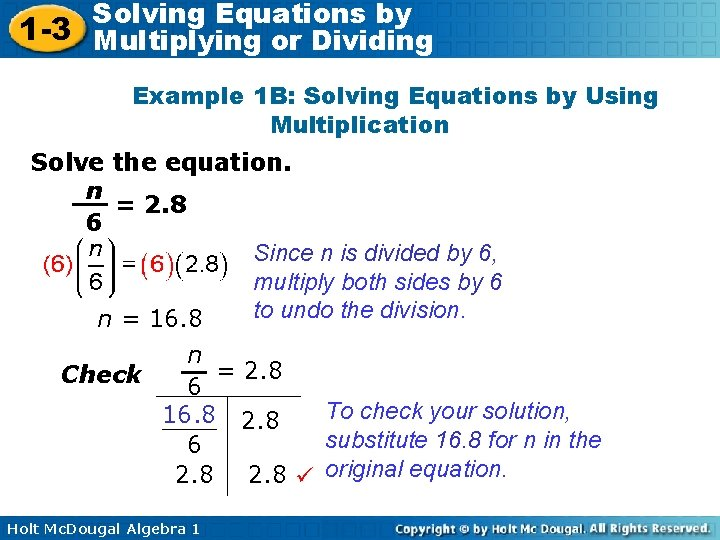Solving Equations by 1 -3 Multiplying or Dividing Example 1 B: Solving Equations by Using Multiplication Solve the equation. n = 2. 8 6 Since n is divided by 6, multiply both sides by 6 to undo the division. n = 16. 8 n = 2. 8 Check 6 To check your solution, 16. 8 2. 8 substitute 16. 8 for n in the 6 2. 8 original equation. Holt Mc. Dougal Algebra 1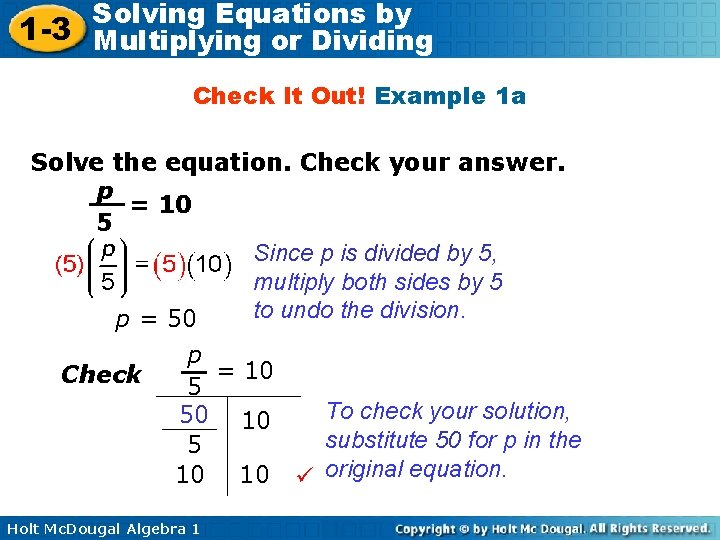Solving Equations by 1 -3 Multiplying or Dividing Check It Out! Example 1 a Solve the equation. Check your answer. p = 10 5 Since p is divided by 5, multiply both sides by 5 to undo the division. p = 50 Check p = 10 5 To check your solution, 50 10 substitute 50 for p in the 5 10 10 original equation. Holt Mc. Dougal Algebra 1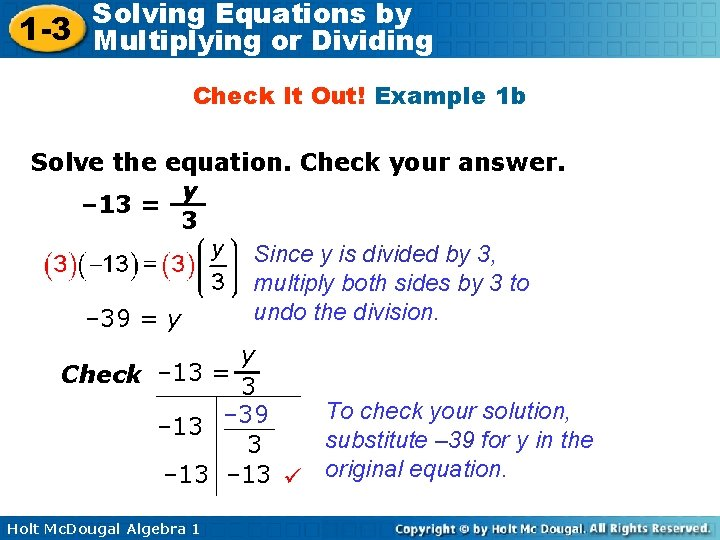Solving Equations by 1 -3 Multiplying or Dividing Check It Out! Example 1 b Solve the equation. Check your answer. y – 13 = 3 Since y is divided by 3, multiply both sides by 3 to undo the division. – 39 = y y Check – 13 = 3 To check your solution, – 39 – 13 substitute – 39 for y in the 3 – 13 original equation. Holt Mc. Dougal Algebra 1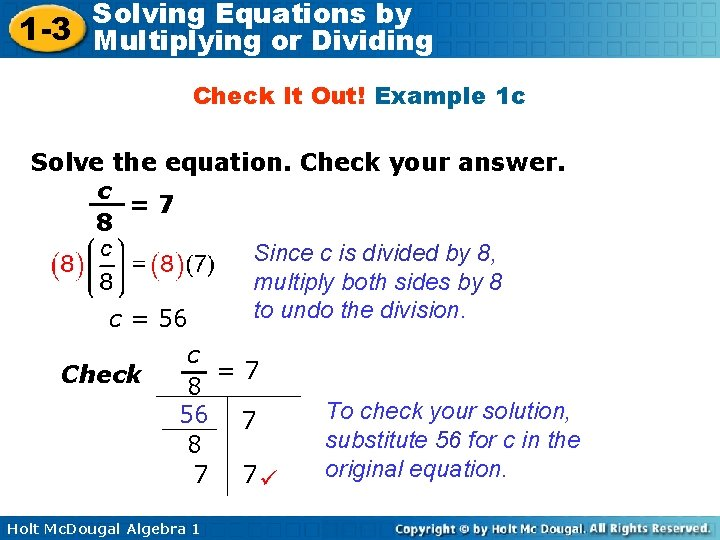Solving Equations by 1 -3 Multiplying or Dividing Check It Out! Example 1 c Solve the equation. Check your answer. c =7 8 Since c is divided by 8, multiply both sides by 8 to undo the division. c = 56 Check c =7 8 56 7 8 7 7 Holt Mc. Dougal Algebra 1 To check your solution, substitute 56 for c in the original equation.Solving Equations by 1 -3 Multiplying or Dividing Example 2 A: Solving Equations by Using Division Solve the equation. Check your answer. 9 y = 108 y = 12 Check Since y is multiplied by 9, divide both sides by 9 to undo the multiplication. 9 y = 108 9(12) 108 To check your solution, substitute 12 for y in the 108 original equation. Holt Mc. Dougal Algebra 1Solving Equations by 1 -3 Multiplying or Dividing Example 2 B: Solving Equations by Using Division Solve the equation. Check your answer. – 4. 8 = – 6 v 0. 8 = v Since v is multiplied by – 6, divide both sides by – 6 to undo the multiplication. Check – 4. 8 = – 6 v – 4. 8 – 6(0. 8) – 4. 8 Holt Mc. Dougal Algebra 1 To check your solution, substitute 0. 8 for v in the original equation.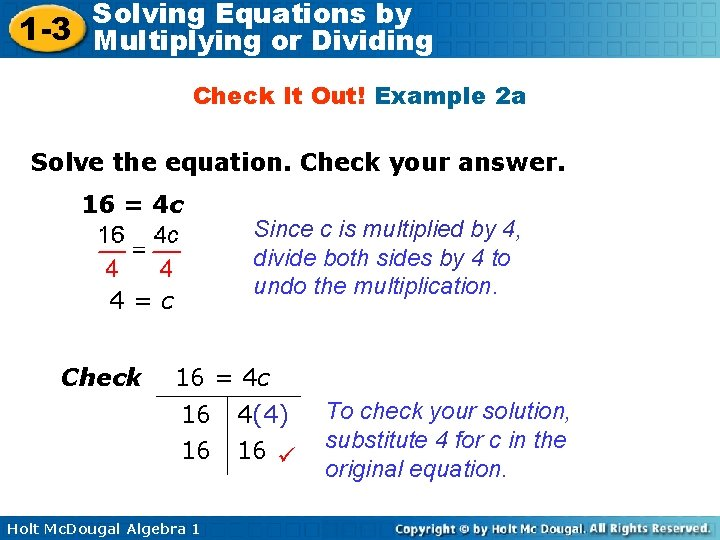Solving Equations by 1 -3 Multiplying or Dividing Check It Out! Example 2 a Solve the equation. Check your answer. 16 = 4 c 4=c Check Since c is multiplied by 4, divide both sides by 4 to undo the multiplication. 16 = 4 c 16 16 Holt Mc. Dougal Algebra 1 4(4) 16 To check your solution, substitute 4 for c in the original equation.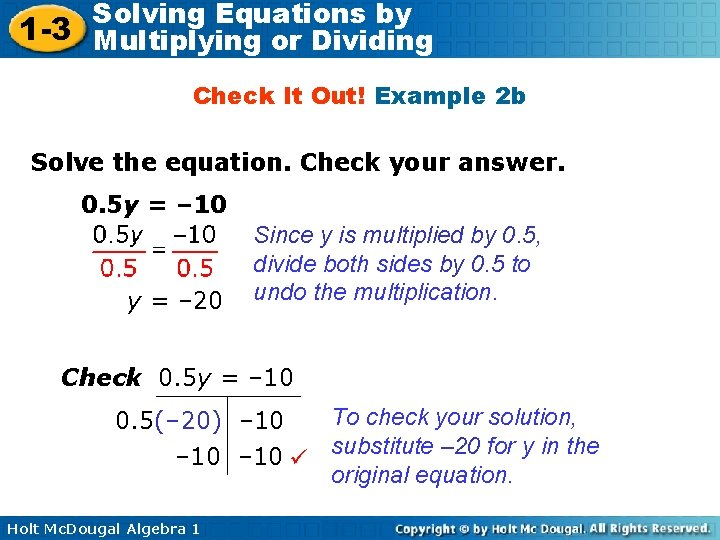Solving Equations by 1 -3 Multiplying or Dividing Check It Out! Example 2 b Solve the equation. Check your answer. 0. 5 y = – 10 y = – 20 Since y is multiplied by 0. 5, divide both sides by 0. 5 to undo the multiplication. Check 0. 5 y = – 10 To check your solution, 0. 5(– 20) – 10 substitute – 20 for y in the original equation. Holt Mc. Dougal Algebra 1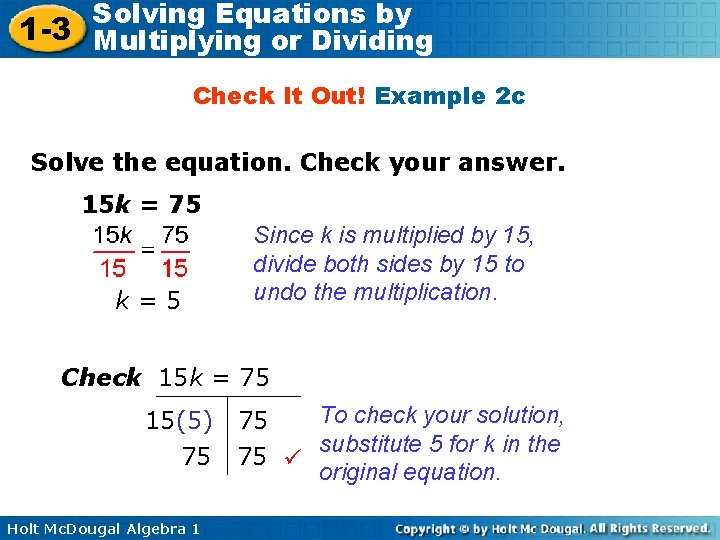Solving Equations by 1 -3 Multiplying or Dividing Check It Out! Example 2 c Solve the equation. Check your answer. 15 k = 75 k=5 Since k is multiplied by 15, divide both sides by 15 to undo the multiplication. Check 15 k = 75 15(5) 75 Holt Mc. Dougal Algebra 1 To check your solution, 75 substitute 5 for k in the 75 original equation.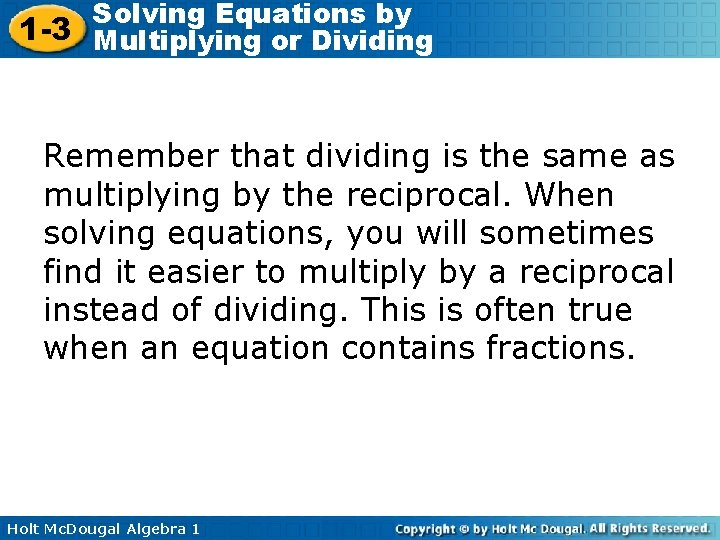Solving Equations by 1 -3 Multiplying or Dividing Remember that dividing is the same as multiplying by the reciprocal. When solving equations, you will sometimes find it easier to multiply by a reciprocal instead of dividing. This is often true when an equation contains fractions. Holt Mc. Dougal Algebra 1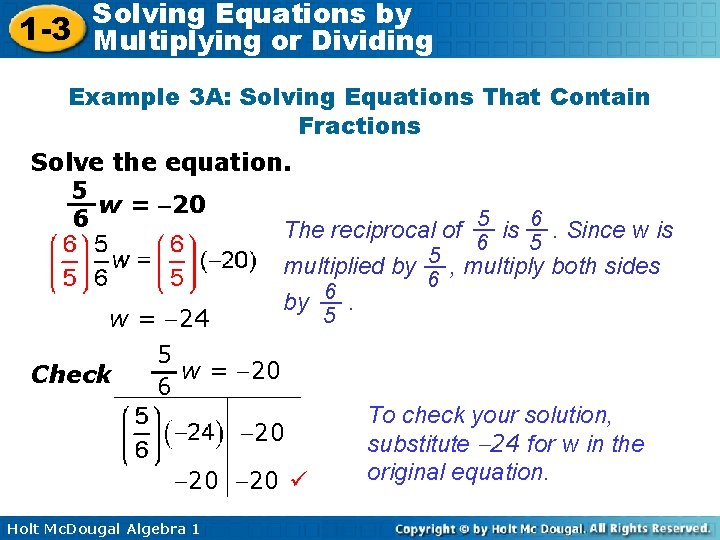Solving Equations by 1 -3 Multiplying or Dividing Example 3 A: Solving Equations That Contain Fractions Solve the equation. 5 w = 20 6 The reciprocal of 5 is 6. Since w is 6 5 5 multiplied by , multiply both sides 6 6 by. 5 w = 24 Check 5 w = 20 6 20 20 20 Holt Mc. Dougal Algebra 1 To check your solution, substitute 24 for w in the original equation.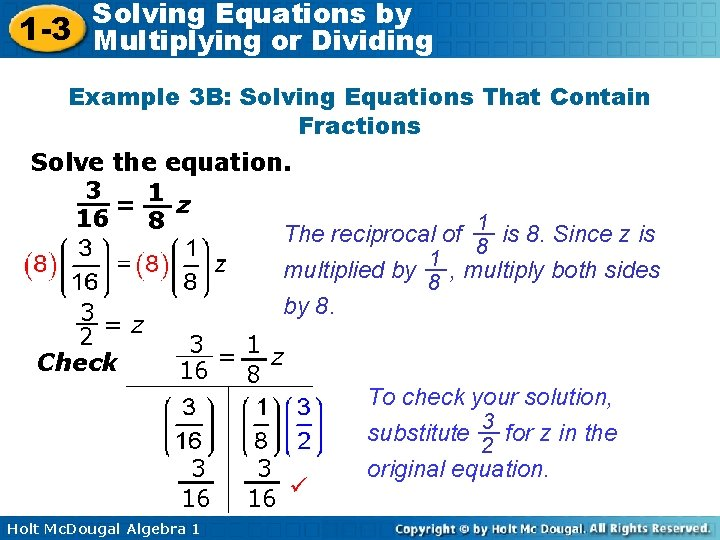Solving Equations by 1 -3 Multiplying or Dividing Example 3 B: Solving Equations That Contain Fractions Solve the equation. 3 = 1 z 16 8 The reciprocal of 1 is 8. Since z is 8 1 multiplied by , multiply both sides 8 by 8. 3=z 2 3 =1 z Check 16 8 To check your solution, substitute 3 for z in the 2 original equation. 3 3 16 16 Holt Mc. Dougal Algebra 1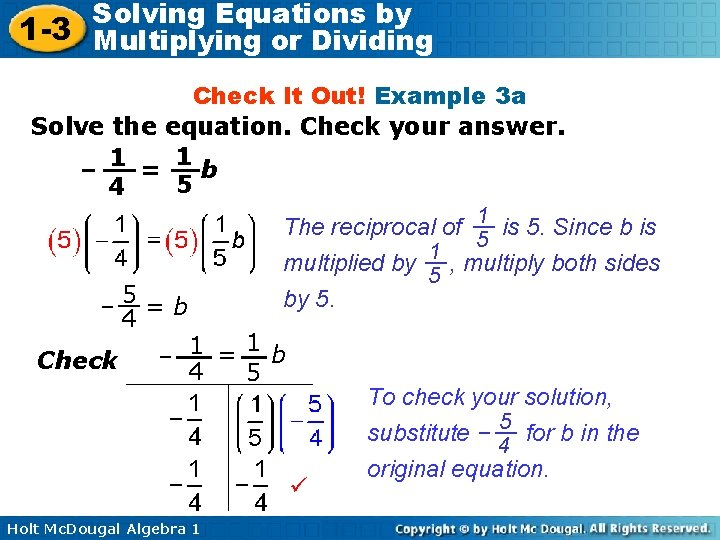Solving Equations by 1 -3 Multiplying or Dividing Check It Out! Example 3 a Solve the equation. Check your answer. 1 – 1 = b 5 4 5=b – 4 Check The reciprocal of 1 is 5. Since b is 5 1 multiplied by , multiply both sides 5 by 5. 1 1 = b – 4 5 Holt Mc. Dougal Algebra 1 To check your solution, substitute – 5 for b in the 4 original equation.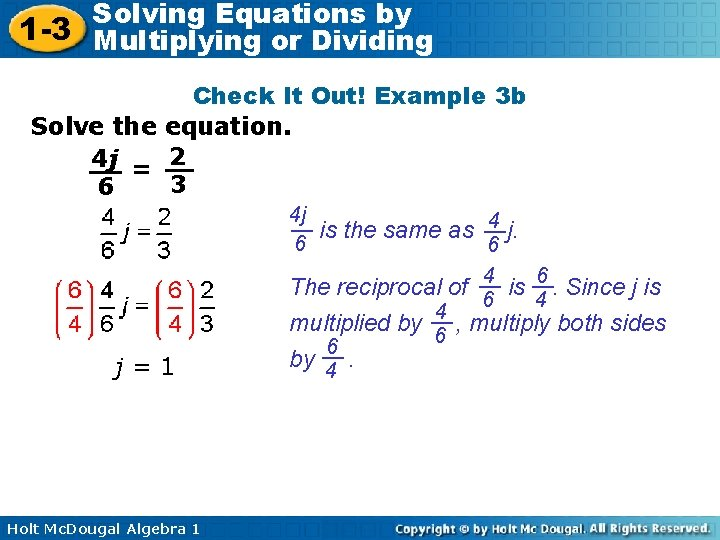Solving Equations by 1 -3 Multiplying or Dividing Check It Out! Example 3 b Solve the equation. 4 j = 2 3 6 j=1 Holt Mc. Dougal Algebra 1 4 j is the same as 4 j. 6 6 The reciprocal of 4 is 6. Since j is 6 4 4 multiplied by , multiply both sides 6 6 by 4.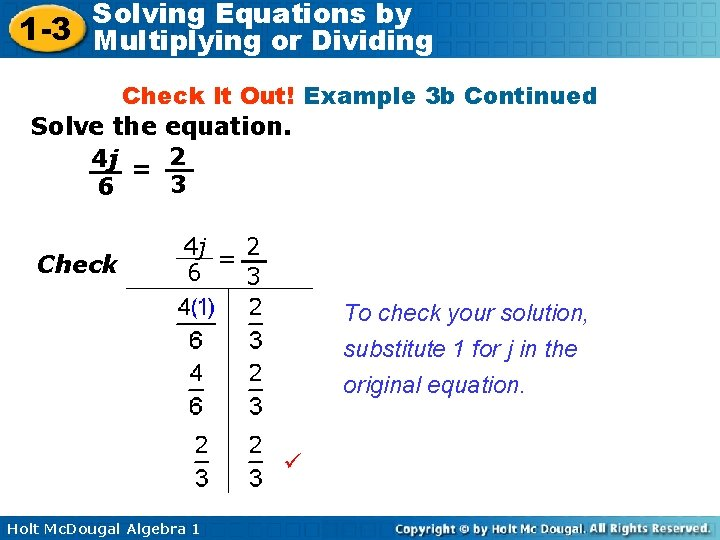Solving Equations by 1 -3 Multiplying or Dividing Check It Out! Example 3 b Continued Solve the equation. 4 j = 2 3 6 Check 2 4 j = 6 3 To check your solution, substitute 1 for j in the original equation. Holt Mc. Dougal Algebra 1Solving Equations by 1 -3 Multiplying or Dividing Check It Out! Example 3 c Solve the equation. 1 w = 102 6 The reciprocal of 1 is 6. Since w is 6 multiplied by 1 , multiply both sides 6 by 6. w = 712 Check 1 w = 102 6 102 102 Holt Mc. Dougal Algebra 1 To check your solution, substitute 712 for w in the original equation.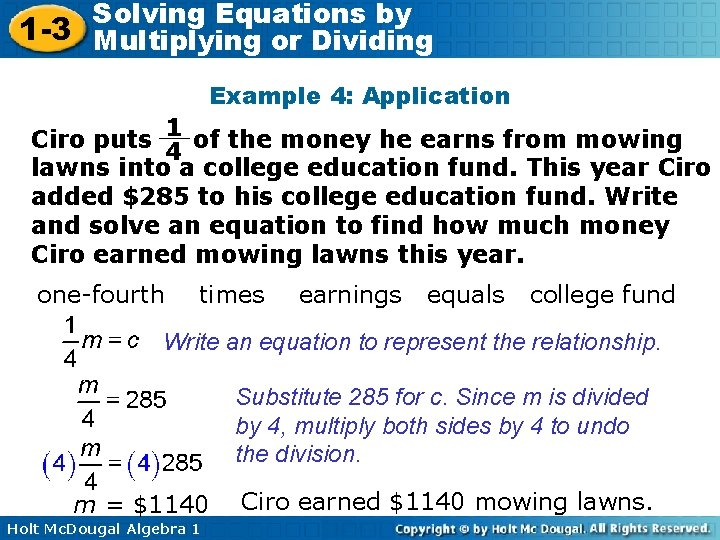Solving Equations by 1 -3 Multiplying or Dividing Example 4: Application 1 Ciro puts 4 of the money he earns from mowing lawns into a college education fund. This year Ciro added \$285 to his college education fund. Write and solve an equation to find how much money Ciro earned mowing lawns this year. one-fourth times earnings equals college fund Write an equation to represent the relationship. Substitute 285 for c. Since m is divided by 4, multiply both sides by 4 to undo the division. m = \$1140 Holt Mc. Dougal Algebra 1 Ciro earned \$1140 mowing lawns.Solving Equations by 1 -3 Multiplying or Dividing Check it Out! Example 4 The distance in miles from the airport that a plane should begin descending, divided by 3, equals the plane's height above the ground in thousands of feet. A plane began descending 45 miles from the airport. Use the equation to find how high the plane was flying when the descent began. Distance divided by 3 equals height in thousands of feet Write an equation to represent the relationship. Substitute 45 for d. 15 = h The plane was flying at 15, 000 ft when the descent began. Holt Mc. Dougal Algebra 1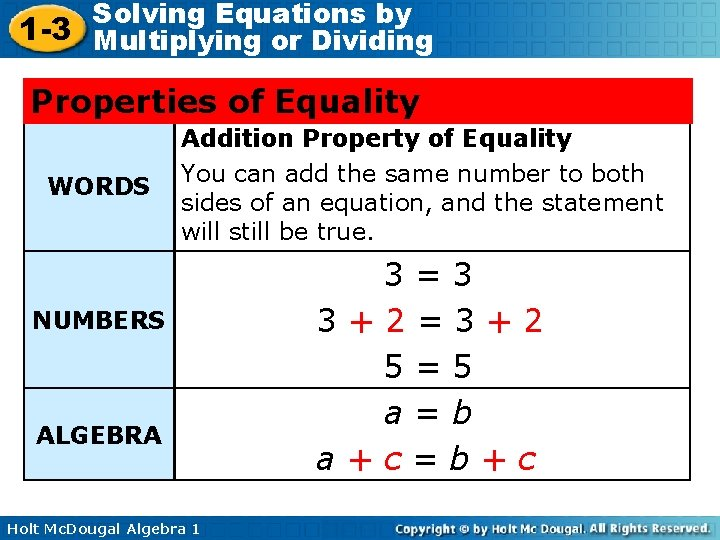Solving Equations by 1 -3 Multiplying or Dividing Properties of Equality WORDS Addition Property of Equality You can add the same number to both sides of an equation, and the statement will still be true. NUMBERS ALGEBRA Holt Mc. Dougal Algebra 1 3=3 3+2=3+2 5=5 a=b a+c=b+c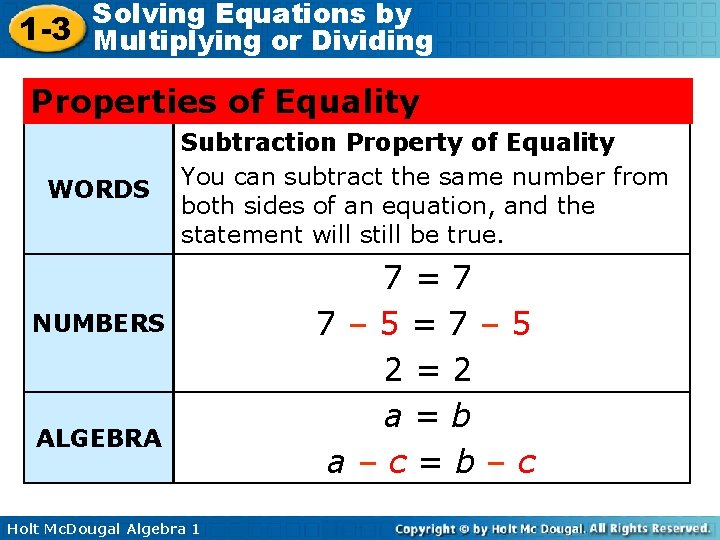Solving Equations by 1 -3 Multiplying or Dividing Properties of Equality WORDS Subtraction Property of Equality You can subtract the same number from both sides of an equation, and the statement will still be true. NUMBERS ALGEBRA Holt Mc. Dougal Algebra 1 7=7 7– 5=7– 5 2=2 a=b a–c=b–cSolving Equations by 1 -3 Multiplying or Dividing Properties of Equality WORDS Multiplication Property of Equality You can multiply both sides of an equation by the same number, and the statement will still be true. NUMBERS ALGEBRA Holt Mc. Dougal Algebra 1 6=6 6(3) = 6(3) 18 = 18 a=b ac = bcSolving Equations by 1 -3 Multiplying or Dividing Properties of Equality WORDS Division Property of Equality You can divide both sides of an equation by the same nonzero number, and the statement will still be true. NUMBERS 8 = 8 8 8 = 4 4 2 =2 ALGEBRA a=b (c ≠ 0) a = a c c Holt Mc. Dougal Algebra 1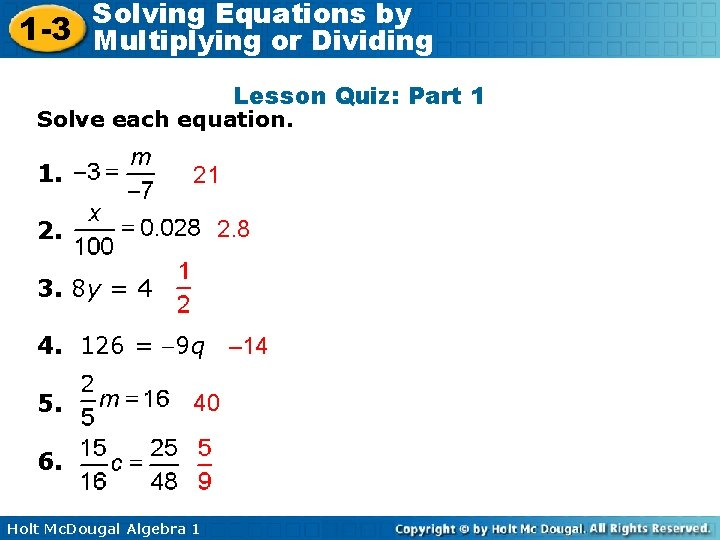Solving Equations by 1 -3 Multiplying or Dividing Lesson Quiz: Part 1 Solve each equation. 1. 21 2. 8 2. 3. 8 y = 4 4. 126 = 9 q – 14 5. 40 6. Holt Mc. Dougal Algebra 1Solving Equations by 1 -3 Multiplying or Dividing Lesson Quiz: Part 2 9 7. A person's weight on Venus is about his or 10 her weight on Earth. Write and solve an equation to find how much a person weighs on Earth if he or she weighs 108 pounds on Venus. Holt Mc. Dougal Algebra 1